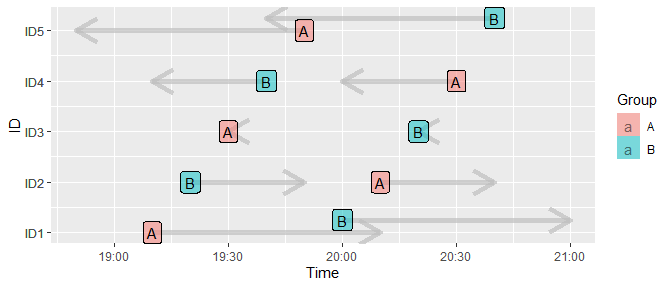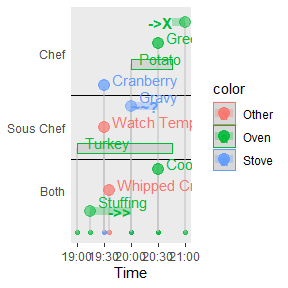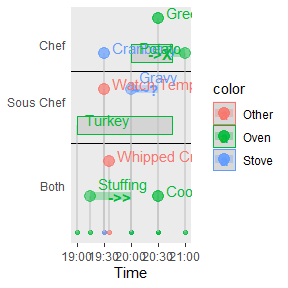# vignette_mtb_time_related

#### 2022-02-04

library(mtb)

## Background

It’s common to have observations that were measured longitudinally. Here are some functions that could present observations measured over time.

## How to use - plot event periods with grouped labels

This is a basic example which shows you how to plot intervals of events with group labels at the beginning of individual intervals.

dt = data.frame( id=paste('ID', c(seq(1,5), seq(1,5)),sep=""), idn=c(seq(1,5),seq(1,5)), start=1800*seq(1,10)/3, end=1800*(seq(1,10)/3+seq(2,-2)), label=rep(c('A','B'),5) )
dt
#>     id idn start  end label
#> 1  ID1   1   600 4200     A
#> 2  ID2   2  1200 3000     B
#> 3  ID3   3  1800 1800     A
#> 4  ID4   4  2400  600     B
#> 5  ID5   5  3000 -600     A
#> 6  ID1   1  3600 7200     B
#> 7  ID2   2  4200 6000     A
#> 8  ID3   3  4800 4800     B
#> 9  ID4   4  5400 3600     A
#> 10 ID5   5  6000 2400     B
p=time_plot_interval( dt, xlab='Time', ylab='ID', legend_title='Group', arrow_wt=2, arrow_color='gray')
p# How to use - create a static timeline plot

There is an existing function vistime::gg_vistime() that creates plots for time periods or single time points. There is also an article by Ben Alex Keen that uses ggplot2 for plotting a series of events.

The time_plot_event() function creates a simplified timeline plot.

dt = data.frame( id=c(rep('Sous Chef',3), rep('Both',3), rep('Chef',4)), idn=c(rep(1,3),rep(-1,3), rep(2,4)), start=1800*c(0,1,2, 0.5, 1.2, 3, 1,2,3,4), end=1800*c(3.5,NA,3, 2, 6, NA, 2,3.5,3, 3.5),
label=c('Turkey', 'Watch Temp', 'Gravy', 'Stuffing', 'Whipped Cream', 'Cookie', 'Cranberry', 'Potato', 'Green Bean', 'Pumpkin Pie'), labelend=c('', '', '~~?', '->>', 'not shown', '', '', '', '||', '->X'), color=c('Oven', 'Other', 'Stove','Oven','Other','Oven','Stove','Oven','Oven','Oven' ), type=c('b', 'p', 'i','i','p','p','p','b','i','i' ) )
dt[1:5,]
#>          id idn start   end         label  labelend color type
#> 1 Sous Chef   1     0  6300        Turkey            Oven    b
#> 2 Sous Chef   1  1800    NA    Watch Temp           Other    p
#> 3 Sous Chef   1  3600  5400         Gravy       ~~? Stove    i
#> 4      Both  -1   900  3600      Stuffing       ->>  Oven    i
#> 5      Both  -1  2160 10800 Whipped Cream not shown Other    p
time_plot_event( dt )Note that the compact option can reduce the use of vertical spaces. However, to avoid reversed interval like event-10, the input data set should have start time < end time.

time_plot_event( dt, compact=TRUE )